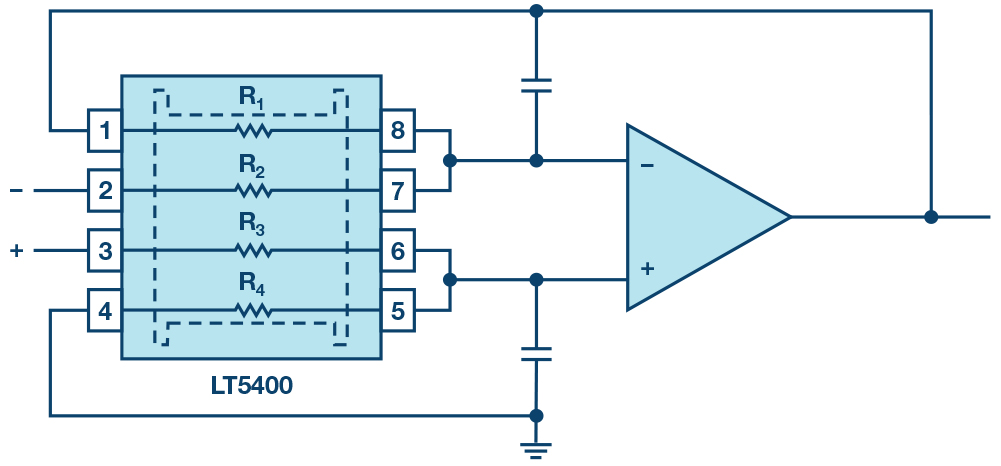## Increasing the common-mode rejection ratio of differential amplifiers

SeriesBy Thomas Brand, Field Applications engineer, Analog Devices

Differential amplifier circuits (Figure 1) are required in many applications; for example, in systems where an extremely high level of measurement accuracy is needed. To achieve this accuracy, it’s important to minimise any error sources, such as offset and gain errors, as well as noise, tolerances and drift. For this purpose, high -precision operational amplifiers are used.

Also important are the external components of the amplifier circuit, especially the resistors, which should have matching ratios rather than be arbitrarily chosen.

Figure 1: Conventional differential amplifier circuit

Ideally, the resistors in differential amplifier circuits should be selected to have identical ratios (R2/R1 = R4/R3), otherwise it may lead to an undesired common-mode error. The ability of a differential amplifier to reject this common-mode error is given in terms of the common-mode rejection ratio (CMRR), which indicates how the output voltage changes with identical input voltages (common-mode voltages). In the best case, the output voltage should not change because it only depends on the difference between the two input voltages (max CMRR). This differs in practice, however.

CMRR is an important characteristic of differential amplifier circuits and is usually given in dB. For differential amplifier circuits such as the one shown in Figure 1, the CMRR is determined by the amplifier itself, as well as the externally-connected resistors. The resistor-dependent CMRR is denoted by “R” in the remainder of this article and is calculated using the following equation:(1)

For example, a desired gain of G = 1 and using resistors with a tolerance of 1% matched to 2% in the amplifier circuit yields a CMRR of:

or in dB:(2)

At 34dB, the CMRRR is relatively low. In this case, even if the amplifier has a very good CMRR, high accuracy can’t be achieved as the chain is always as strong as its weakest link.

Precisely measured

Accordingly, for precise measuring circuits, the selected resistors must also be extremely precise.

Conventional resistors don’t have constant values in practice because of the mechanical loads and effects of temperature they are subjected to. Depending on the requirement, resistors with different tolerances or matched resistor pairs or networks may be used, which for the most part are made using thin-film technology and offer precise ratiometric stability. With these matched resistor networks (for example, the LT5400 quad matched resistor network), the overall CMRR of the amplifier circuit can be dramatically improved. The LT5400 resistor network guarantees a CMRR up to two times better compared with discrete resistors solutions, due to its outstanding matching over the entire temperature range, especially when used in combination with differential amplifier circuits.

Figure 2: Differential amplifier circuit with LT5400

Thus, the LT5400 offers 0.005% matching, which results in a CMRRR of 86dB.

However, the total common-mode rejection ratio of amplifier circuits (CMRRTotal) is formed by the combination of the resistor CMRR and the common-mode rejection ratio of the op-amp, CMRROP . For differential amplifiers, this is calculated using Equation 3:(3)

For a typical CMRROP of 112dB, as offered by the LT1468, and a gain of G = 1 with the LT5400, a value of 85.6dB is yielded for CMRRTotal.

Alternatively, integrated differential amplifiers such as the LTC6363 can be used, a solution that already contains the actual amplifier and optimally-matched resistors on a chip. It eliminates nearly all the problems mentioned here, and offers maximum accuracy at CMRR values of over 90dB.

##### Accuracy requirements

The external resistor circuit must be selected carefully based on the requirements for accuracy of the differential amplifier circuit, to achieve that high system performance.

Alternatively, integrated differential amplifiers such as the LTC6363 with integrated matched resistors on-chip can also be used.